Patterning is one of the basic tasks of designing tensile membrane structures. It is also one of three modules of WinTess software shown in top bar. The usual way to transform a warped curved surface in flat strips (patterns) is doing by triangulation. It is the easiest and most used way.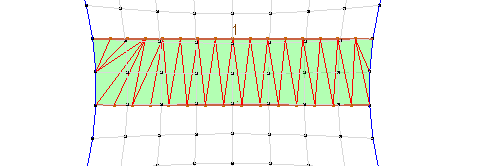Typical pattern

In another post in this blog (cross compensation) we already discussed triangulation of patterns.
Given that membrane is delivered in long rolls, the most common approach is also to create long patterns. To do this, we often draw two roughly parallel geodesic lines and triangulate as shown below.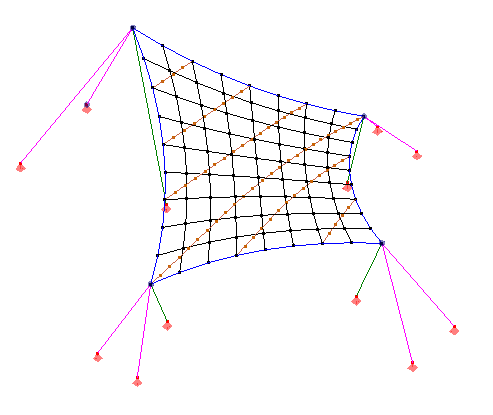Geodesic lines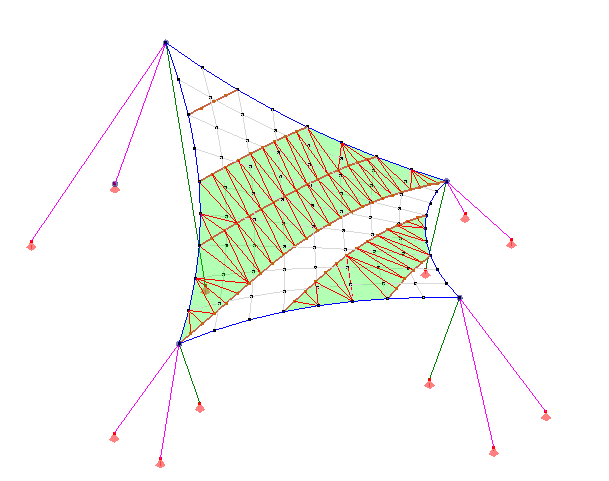Triangularization

WinTess provides a manual method (triangles) and two automatic methods (between two geodesic, by perimeter) for triangulation. However, any regular user of the program will be found in situations where a quick triangulation is not possible. We will shortly consider a number of those typical cases. No doubt that they will be many others which do not not match any of the mentioned here. This only supports the assertion that it is impossible to consider all actual cases that may come up.

1. Simple pattern

The simplest possible pattern is located between two roughly parallel geodesic lines with same number of nodes and begin and end much the same. It is a very easy case of triangulation which can be done by WinTess automatically (two geodesic). However, check that the ends of the pattern matches the edge of the membrane.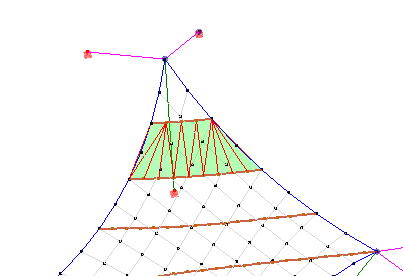Simple pattern

2. Pattern “quasi” simple

This is perhaps the most common case. There are two roughly parallel geodesic, but it is not a straight line between their ends, instead there is a piece of perimeter of the membrane. If one geodesic is clearly longer than the other at one end, triangulation remains quite easy and also WinTess can make it automatically (perimeter).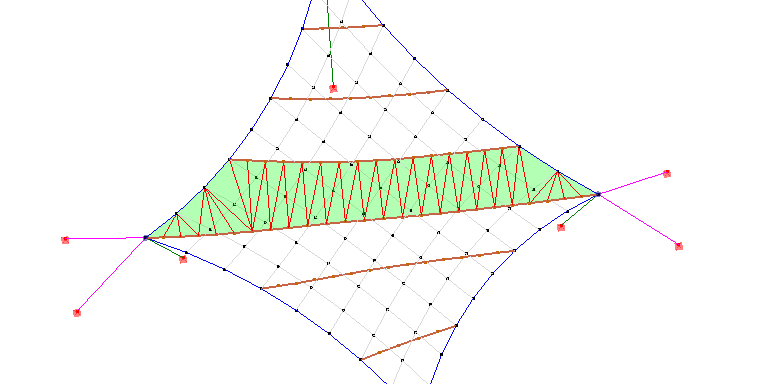Pattern quasi simple

3. Pattern with peaks at the ends

This is a pattern between two roughly parallel geodesic lines with ends that form spikes due to the shape of the membrane itself. In fact it is as if they were two geodesic that have continued to meet at one point. It remains an easy task of triangulation and pattern can be created automatically with WinTess (for perimeter).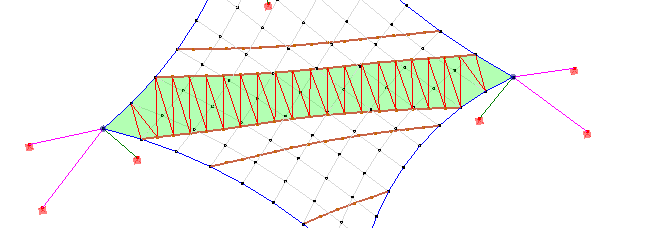Pattern with peaks

4. Pattern with convex end

This is a kind of pattern between two roughly parallel geodesic lines ending without a clear membrane edge, as happened in the previous case. In general it begins to be a somewhat complex pattern, as it surely will force us to make a series of very sharp triangles that are not generally desirable for pattern quality. Whether we do it manually (by triangles) or automatically (by perimeter) it is advisable to perform a check on the quality of the pattern after generating it.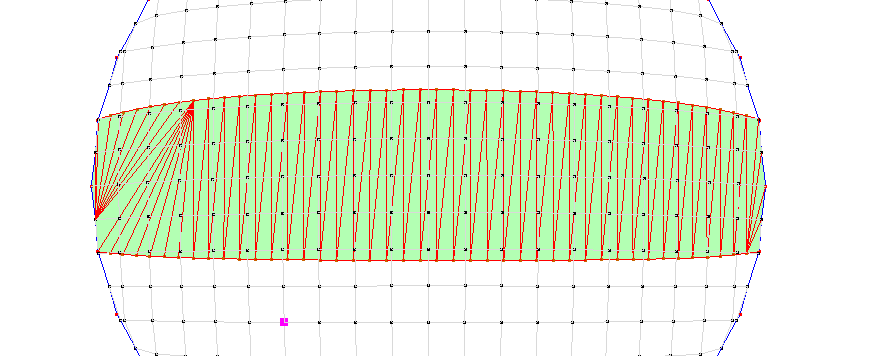Ends with convex pattern

In the previous example of the pattern, we see how creating a pattern ” for perimeter ” method by WinTess is not the same way of triangulation on both sides. Surely the way to do it on the right side is better than the left. In most of these cases we will have to make the pattern manually using ” triangles ” method.

5. Pattern with concave end

This is actually a fairly common case because of the curvature of the edges of a membrane. The ends between the two geodesic lines normally create a pattern forming inward curves creating a recess on the outside. If the curve is very soft (it is almost a straight), patterning can be similar to the previous case.Concave pattern ends

We see that triangulation can be done in various ways at the ends. The shape on the right seems better than the left one. In this example we have used the automatic method ” for perimeter “.  Now if the depression is very pronounced there is no way to find a satisfactory simple triangulation. Suppose this membrane: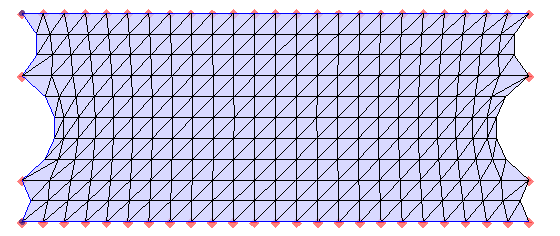Concavities at pattern ends

and a pattern like this: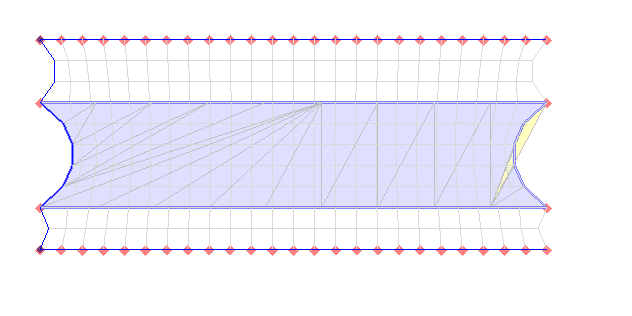Concave pattern at the ends

This pattern was created using the method “for perimeter”. We see on the left side that triangles had to be made from the center to keep the concavity. On the right, however, to make the most logical triangles, it has crossed the perimeter and created an impossible triangle. Obviously, we conclude that patterns cannot be solved in a simple way with concave patterns. So, we have to force to solve the problem by using a composite pattern or ” negative patterns “. What is a negative pattern? It is a pattern to be subtracted from a base pattern to get the actual pattern.

In the same example above we can make a base pattern between the two geodesic lines and get the following: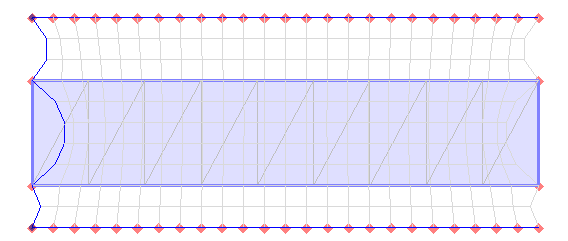Previous pattern (between 2 geodesics)

and then we create the ” negative patterns ” at two ends: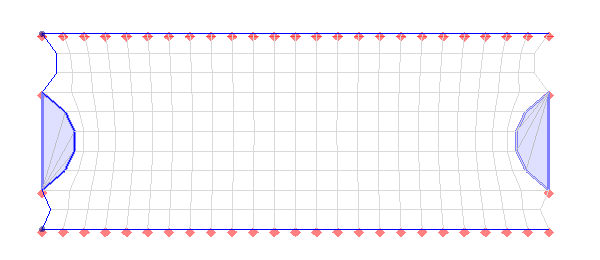“Negative patterns” at ends

That way we get three patterns. Once exported as DXF, we can put negative patterns over the base pattern using our usual CAD program and obtain the final pattern.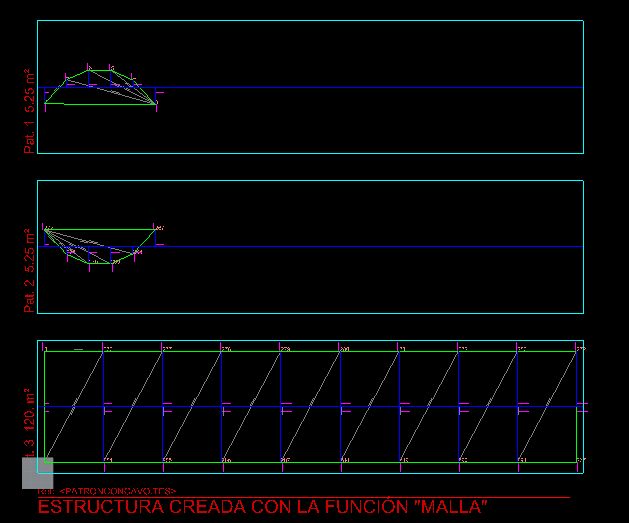Patterns in DXF

Here is the result: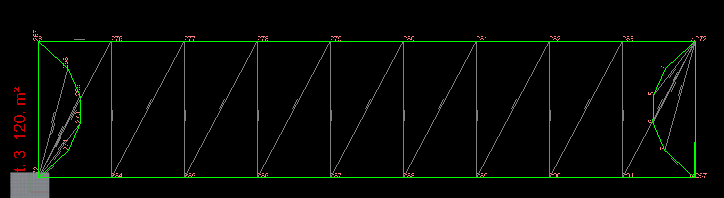Final pattern

As you can see, it is not a simple process.

6. Pattern with many interior points

There are times that we use a very dense mesh with many elements for having a smoother appearance. In the manual of WinTess already discussed Mesh Density  What is a correct mesh or a mesh too dense? Well, what interests us here is to comment that too many interior nodes due to a dense mesh are useless. Pattern uses only the perimeter points for its creation.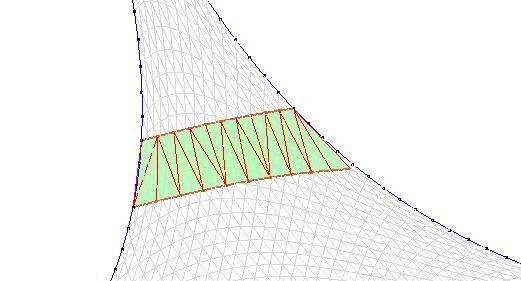Pattern with very dense mesh

Moreover, it is likely that when we check quality of pattern we will be surprised to see that WinTess considers it as of poor quality, as it moves away from the interior nodes.

We can say, somehow, that the mesh size should not be more than the pattern needs. Overall the mesh size should not be less than one third of the width of the pattern: with a membrane of 180 cm wide, the mesh should not be more than 60 cm.

7. Pattern with no longitudinal

So far we have always been talking about patterns between geodesic lines and ends. It is the most typical case, but not unique. Let’s see what happens when the ends or “short” sides are of the same length of “long” sides.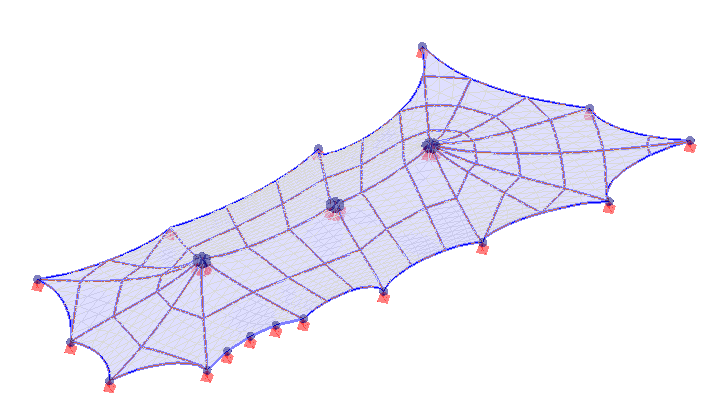Pattern with no longitudinal

In these cases it is very difficult to create a pattern by triangulation. It does not quite know where to start, as there is no long side or short side. It is clear that to get from one side to the other will lose all the information on the screen that is inside (as we discussed in the previous section 6). Sometimes  we can make a diagonal triangulation, but it is not going to be a good solution.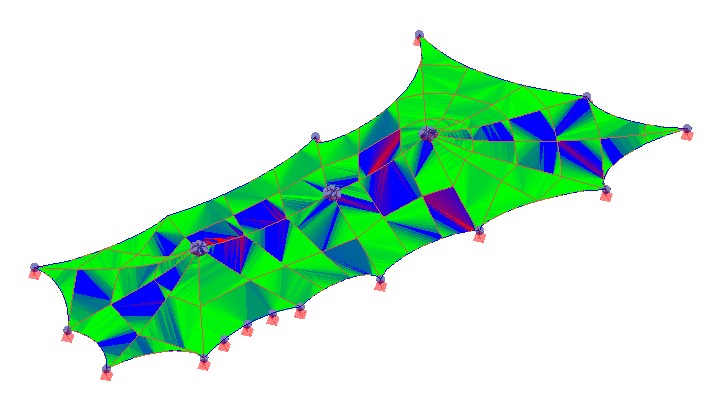Pattern quality (see the diagonal)

We can always try to improve the resolution of the pattern through the cross compensation. But this is merely a way of trying to solve a difficult pattern that needs to be avoided best.